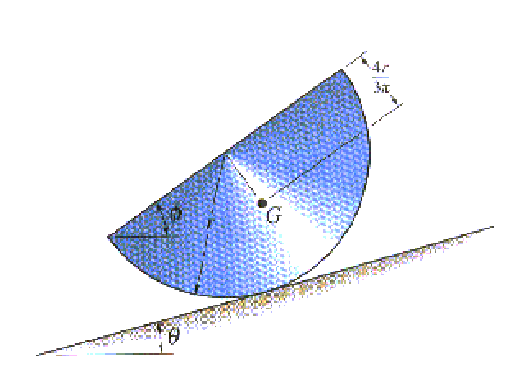Statics-Sample Exam III

Note to the students: The following is a typical "Exam III" for statics. It should give you an idea of the difficulty of the exam. Try to do the exam in 50 minutes without the aid of your book or your notes, only using your one page of equations (no solutions to problems). For each problem, first layout the procedure to obtain the desired solution, then complete the problem by doing all the details. Don't forget to draw free-body diagrams for the equilibrium problems, and to present your result in three significant digits with proper units. If the figures are not clear, you can find them in the book.

Problem 1: Determine the force in members BC, FC, and FE and state if these members are in tension or compression.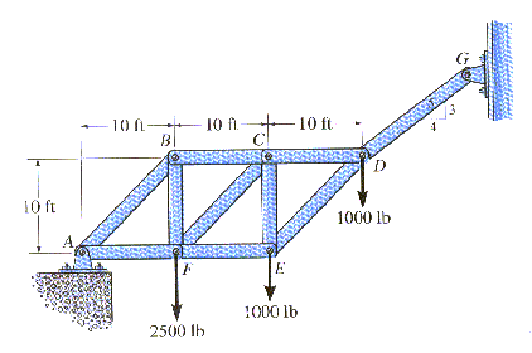Problem 2: Determine the normal force, shear force, and moment at a section passing through point D of the two-member frame.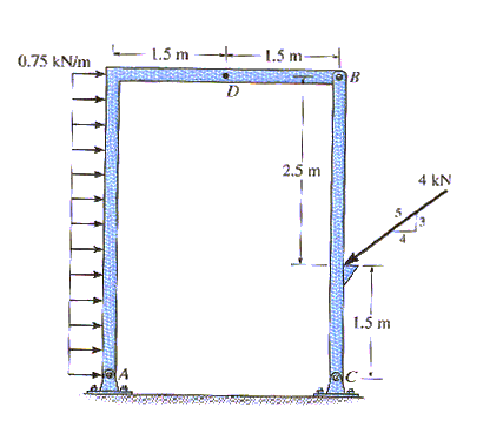Problem 3: The homogeneous semicylinder has a mass of m and mass center at G. Determine the largest angle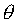of the inclined plane upon which it rests so that it does not slip down the plane. The coefficient of static friction between the plane and the cylinder is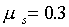. Also, what is the anglefor this case.